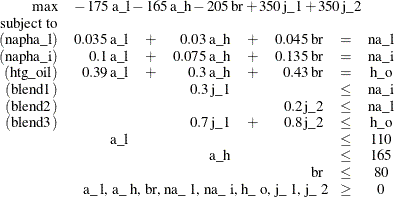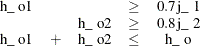### Example 10.1 Oil Refinery Problem

Consider an oil refinery scenario. A step in refining crude oil into finished oil products involves a distillation process that splits crude into various streams. Suppose there are three types of crude available: Arabian light (a_l), Arabian heavy (a_h), and Brega (br). These crudes are distilled into light naphtha (na_l), intermediate naphtha (na_i), and heating oil (h_o). These in turn are blended into two types of jet fuel. Jet fuel j_1 is made up of 30% intermediate naphtha and 70% heating oil, and jet fuel j_2 is made up of 20% light naphtha and 80% heating oil. What amounts of the three crudes maximize the profit from producing jet fuel (j_1, j_2)? This problem can be formulated as the following linear program:The constraints blend1 and blend2 ensure that j_1 and j_2 are made with the specified amounts of na_i and na_l, respectively. The constraint blend3 is actually the reduced form of the following constraints:where h_o1 and h_o2 are dummy variables.

You can use the following SAS code to create the input data set ex1:

data ex1;
input field1 $field2$ field3 $field4 field5$ field6;
datalines;
NAME        .          EX1      .     .         .
ROWS        .          .        .     .         .
N          profit     .        .     .         .
E          napha_l    .        .     .         .
E          napha_i    .        .     .         .
E          htg_oil    .        .     .         .
L          blend1     .        .     .         .
L          blend2     .        .     .         .
L          blend3     .        .     .         .
COLUMNS     .          .        .     .         .
.           a_l        profit   -175  napha_l   .035
.           a_l        napha_i  .100  htg_oil   .390
.           a_h        profit   -165  napha_l   .030
.           a_h        napha_i  .075  htg_oil   .300
.           br         profit   -205  napha_l   .045
.           br         napha_i  .135  htg_oil   .430
.           na_l       napha_l  -1    blend2    -1
.           na_i       napha_i  -1    blend1    -1
.           h_o        htg_oil  -1    blend3    -1
.           j_1        profit   350   blend1    .3
.           j_1        blend3   .7    .         .
.           j_2        profit   350   blend2    .2
.           j_2        blend3   .8    .         .
BOUNDS      .          .        .     .         .
UP          .          a_l      110   .         .
UP          .          a_h      165   .         .
UP          .          br       80    .         .
ENDATA      .          .        .     .         .
;


You can use the following call to PROC OPTLP to solve the LP problem:

proc optlp data=ex1
objsense  = max
algorithm = primal
primalout = ex1pout
dualout   = ex1dout
logfreq   = 1;
run;
%put &_OROPTLP_;


Note that the OBJSENSE=MAX option is used to indicate that the objective function is to be maximized.

The primal and dual solutions are displayed in Output 10.1.1.

Output 10.1.1: Example 1: Primal and Dual Solution Output

 Primal Solution

Obs Objective Function ID RHS ID Variable
Name
Variable
Type
Objective
Coefficient
Lower
Bound
Upper Bound Variable Value Variable
Status
Reduced Cost
1 profit   a_l D -175 0 110 110.000 U 10.2083
2 profit   a_h D -165 0 165 0.000 L -22.8125
3 profit   br D -205 0 80 80.000 U 2.8125
4 profit   na_l N 0 0 1.7977E308 7.450 B 0.0000
5 profit   na_i N 0 0 1.7977E308 21.800 B 0.0000
6 profit   h_o N 0 0 1.7977E308 77.300 B 0.0000
7 profit   j_1 N 350 0 1.7977E308 72.667 B 0.0000
8 profit   j_2 N 350 0 1.7977E308 33.042 B 0.0000
 Dual Solution

Obs Objective Function ID RHS ID Constraint Name Constraint
Type
Constraint
RHS
Constraint
Lower
Bound
Constraint
Upper
Bound
Dual Variable
Value
Constraint
Status
Constraint Activity
1 profit   napha_l E 0 . . 0.000 L 0.00000
2 profit   napha_i E 0 . . -145.833 U 0.00000
3 profit   htg_oil E 0 . . -437.500 U 0.00000
4 profit   blend1 L 0 . . 145.833 L 0.00000
5 profit   blend2 L 0 . . 0.000 B -0.84167
6 profit   blend3 L 0 . . 437.500 L 0.00000

The progress of the solution is printed to the log as follows.

Output 10.1.2: Log: Solution Progress

 NOTE: The problem EX1 has 8 variables (0 free, 0 fixed). NOTE: The problem has 6 constraints (3 LE, 3 EQ, 0 GE, 0 range). NOTE: The problem has 19 constraint coefficients. WARNING: The objective sense has been changed to maximization. NOTE: The LP presolver value AUTOMATIC is applied. NOTE: The LP presolver removed 3 variables and 3 constraints. NOTE: The LP presolver removed 6 constraint coefficients. NOTE: The presolved problem has 5 variables, 3 constraints, and 13 constraint coefficients. NOTE: The LP solver is called. NOTE: The Primal Simplex algorithm is used. Objective                Entering      Leaving Phase Iteration        Value         Time     Variable      Variable P 1          1    0.000000E+00         0 P 2          2    0.000000E+00         0        j_1         blend1 (S) P 2          3    1.405640E-01         0        j_2         blend3 (S) P 2          4    1.454487E-01         0        a_l         blend2 (S) P 2          5    2.379819E-01         0         br            a_l P 2          6    1.202394E+03         0     blend2 (S)         br P 2          7    1.348074E+03         0 D 2          8    1.347917E+03         0 D 2          9    1.347917E+03         0 NOTE: Optimal. NOTE: Objective = 1347.9166667. NOTE: The Primal Simplex solve time is 0.03 seconds. NOTE: The data set WORK.EX1POUT has 8 observations and 10 variables. NOTE: The data set WORK.EX1DOUT has 6 observations and 10 variables.

Note that the %put statement immediately after the OPTLP procedure prints value of the macro variable _OROPTLP_ to the log as follows.

Output 10.1.3: Log: Value of the Macro Variable _OROPTLP_

 STATUS=OK   ALGORITHM=PS   SOLUTION_STATUS=OPTIMAL   OBJECTIVE=1347.9166667 PRIMAL_INFEASIBILITY=3.552714E-15   DUAL_INFEASIBILITY=0 BOUND_INFEASIBILITY=0   ITERATIONS=9   PRESOLVE_TIME=0.00   SOLUTION_TIME=0.03

The value briefly summarizes the status of the OPTLP procedure upon termination.